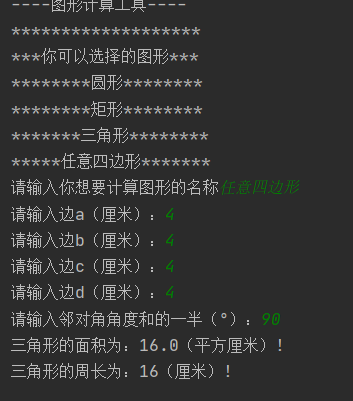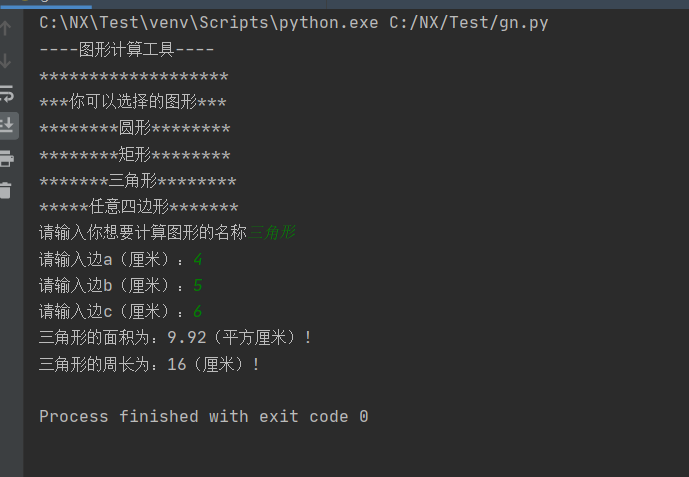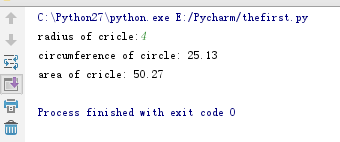• #Python3 实例–Python 计算圆的面积 print(“Python3 实例–Python 计算圆的面积”) #公式中 r 为圆的半径。 r = float(input()) PI = 3.14 s = PI * ( r ** 2 ) print(“圆的面积为：{}”.format(s)) #运行结果...
#代码如下：
#Python3 实例–Python 计算圆的面积
print(“Python3 实例–Python 计算圆的面积”)
#公式中 r 为圆的半径。
r = float(input())
PI = 3.14
s = PI * ( r ** 2 )
print(“圆的面积为：{}”.format(s))
#运行结果如下：
Python3 实例–Python 计算圆的面积
3
圆的面积为：28.26


展开全文• Python编写关于计算图形面积的代码实现 小白入手第一次发表CSDN，不怎么会用，代码也写的比较简单，请各位大佬多多指教谢谢 #寒假打卡28天第7天 import math class Round(): #圆形 def __init__(self, R): ...
用Python编写关于计算图形面积的代码实现
小白入手第一次发表CSDN，不怎么会用，代码也写的比较简单，请各位大佬多多指教谢谢
#寒假打卡28天第7天
import math

class Round(): #圆形
def __init__(self, R):
self.R = R

def area(self):
return self.R * self.R * 3.14

def girth(self):
return self.R * 2 * 3.14

class Rectangle():#矩形
def __init__(self, length, width):
self.length = length
self.width = width

def area(self):
return self.length * self.width

def girth(self):
return self.length + self.width

class Triangle():#三角形
def __init__(self, a, b, c):
self.a = a
self.b = b
self.c = c

def area(self):
l = (self.a + self.b + self.c)/2
return round(math.sqrt(l * (l - self.a) * (l - self.b) * (l - self.c)), 2)  #round保留2为小数

def girth(self):
return self.a + self.c + self.c

class Guadrilateral():#任意四边形
def __init__(self, a, b, c, d, angle):
self.a = a
self.b = b
self.c = c
self.d = d
self.angle = angle

def area(self):
l = (self.a + self.b + self.c + self.d)/2
return round(math.sqrt((l - self.a) * (l - self.b) * (l - self.c) * (l - self.d) -
self.a * self.b * self.c * self.d
* math.pow(math.cos(math.radians(self.angle)), 2)), 2) #pow函数幂次方radians弧度转换为角度

def girth(self):
return self.a + self.b + self.c + self.d

print("----图形计算工具----")
print("*******************")
print("***你可以选择的图形***")
print("********圆形********")
print("********矩形********")
print("*******三角形********")
print("*****任意四边形*******")
target = str(input("请输入你想要计算图形的名称"))
if target == "圆形":
x1 = int(input("请输入半径（厘米）："))
print("圆形的面积为：" + str(Round(x1).area()) + "（平方厘米）!")
print("圆形的周长为：" + str(Round(x1).girth()) + "（厘米）!")
elif target == "矩形":
x1 = int(input("请输入长度（厘米）："))
x2 = int(input("请输入宽度（厘米）："))
print("矩形的面积为：" + str(Rectangle(x1, x2).area()) + "（平方厘米）!")
print("矩形的周长为：" + str(Rectangle(x1, x2).girth()) + "（厘米）!")
elif target == "三角形":
x1 = int(input("请输入边a（厘米）："))
x2 = int(input("请输入边b（厘米）："))
x3 = int(input("请输入边c（厘米）："))
print("三角形的面积为：" + str(Triangle(x1, x2, x3).area()) + "（平方厘米）!")
print("三角形的周长为：" + str(Triangle(x1, x2, x3).girth()) + "（厘米）!")
elif target == "任意四边形":
x1 = int(input("请输入边a（厘米）："))
x2 = int(input("请输入边b（厘米）："))
x3 = int(input("请输入边c（厘米）："))
x4 = int(input("请输入边d（厘米）："))
x5 = int(input("请输入邻对角角度和的一半（°）："))
print("三角形的面积为：" + str(Guadrilateral(x1, x2, x3, x4, x5).area()) + "（平方厘米）!")
print("三角形的周长为：" + str(Guadrilateral(x1, x2, x3, x4, x5).girth()) + "（厘米）!")展开全文• # All rightsreserved #文件名称：a.py ...#问题分析：计算圆的周长和面积需要使用π值，Python的math模块中包含常量pi，通过导入math模块可以直接使用该值，然后使用周长和面积公式计算即可。代码如下： imp
#Copyright (c)2017, 东北大学软件学院学生
# All rightsreserved
#文件名称：a.py
# 作    者：孔云
#问题描述：编写程序，从键盘输入圆的半径，计算并输出圆的周长和面积。
#问题分析：计算圆的周长和面积需要使用π的值，Python的math模块中包含常量pi，通过导入math模块可以直接使用该值，然后使用周长和面积公式计算即可。代码如下：
import math
radius=input("radius of cricle:")
circumference=2*math.pi*radius
area=math.pi*radius*radius
print ( "circumference of circle: %.2f" % circumference)
print ( "area of cricle: %.2f"% area)


运行结果：注：初识Python，体检一下它的math模块。

展开全文• 计算圆的周长和面积问题描述问题分析代码实例样例输入 问题描述 设计一一个小程序，运用本节介绍操作运算符实现输人、输出圆形基本 参数。首先了解圆形基本计算公式，如（2-1）所示: C=2r, S=πr^2 ( 2-1) ...
计算圆的周长和面积问题描述问题分析代码实例样例输入
问题描述
设计一一个小程序，运用本节介绍的操作运算符实现输人、输出圆形的基本
参数。首先了解圆形的基本计算公式，如（2-1）所示:
C=2r, S=πr^2    ( 2-1)
其中，r代表圆形的半径，C代表圆形的周长，S代表圆形的面积，π是圆周率。
问题分析

输人半径，输出面积及周长。

注：要求输入框中只能为数字，若输入其他内容，运行后显示输入出错，而非报错代码。
代码实例
r=input('请输入圆的半径：')
if len(r) == 0:
print('输入无效内容')
else:
if r.strip().isdigit():
r=float(r)
PI =3.14
c=2*PI*r
s= PI*(r**2)
print('圆的周长为：',c)##输出圆的周长
print('圆的面积为：',s)##输出圆的面积
else:
print('请检查输入内容是否为数值型，并重新运行程序输入半径')


样例输入
(1)输入框中没有内容输入(2)  r=_10(3)  r=a(4) r= 6
**r=空格61、len()函数判断输入框中内容的长度；确定是否有内容输入；
2、strip()函数能够自动删除数字左右两边的空格；


展开全文• Python练习---PTA程序设计辅助教学平台题目输入格式:输出格式:输入样例:输出样例:问题分析：代码： 题目 输入圆半径计算圆周长、圆面积、...首先我们要了解数学上圆周长、圆面积、圆球表面积的计算公式 圆周长：c=2πr算法
• Python圆面积周长 要求: 输入圆半径r，计算面积(area)和周(circumference)。 注意: 所有数保留两位小数。π取值3.14。方法为：print(“%.2f” % a)。 # 请使用 input() 输入圆半径 r pi = 3.14 r = float...
• 根据用户提供半径，来实现对圆的面积与周长计算。 运用math模块，math.pi得到圆周率 根据用户提供半径，公式计算面积和周长 1.引入库 代码如下（示例）： import math pai = math.pi radius = eval(input('请...
• 代码： r=29 area = 3.1415*r*r print(area) print("{:.2f}".format(area)) 结果：
• 1.计算圆的周长，面积 2.分析： 3.代码： 1.计算圆的周长，面积 2.分析： 圆周率：pi  引入math包里面pi模块  from math import pi 周长：l=2*pi*r 面积：s=pi*r**2 s=pi*r*r 3.代码： ''' ...
• ## 圆面积的计算 S

千次阅读 2017-12-15 16:52:58
描述根据圆半径计算圆面积，半径为25。请编写并运行如下代码：radius = 25 #圆半径是25 area = 3.1415 * radius * radius #根据公式计算面积 print("{:.2f}".format(area)) #仅输出两位小数结果《Python语言...
• 输入半径，计算圆的周长、面积、球体体积，并画出这个。拖动条、输入框和图像控件数据保持一致！ Fedora下测试通过 复制代码 代码如下:#...
• 编写程序，根据输入半径，计算圆的面积。 ''' import math while True: r = input("请输入圆的半径（米）： ") try: r = float(r) area = pow(r,2) * math.pi print(f"该圆的面积为：{area : .2f} 平方...
• 题目二：输入圆的半径计算周长面积 题目要求：输入圆的半径，输出圆的周长和面积。输入输出均为整数或者浮点数 题目分析： 首先使用关键字import导入math数学函数库 获取到用户输入参数（圆的半径） 将用户输入...
• 编写程序，创建类Mymath,计算圆的周长和面积，并编写测试代码。 class Circle: def __init__(self,r): self.rr=r def area(self): self.ar=self.rr*self.rr print('面积是：',self.ar) def zc(self): self.z=...
• Python几何形状区域 这是一个使用Python为学校作业分配程序。该项目目的是开发代码，以使用用户给定输入来计算矩形，三角形和圆形的面积
• 设计一个 Circle 类来表示，这个类包含的半径以及求周长和面积的函 数。再使用这个类创建半径为 1~100 的，并计算出相应的周长及面积。 代码如下： class Circle: r=float(input("请输入的半径1~100：")) ...算法
• 本程序通过一个while循环分两步计算图形面积，...shape = input("请输入要计算面积的图形：（矩形，正方形，三角形，梯形，圆形）") if (shape == "正方形") or (shape == "矩形"): a = int(input("请输入长："))
• 文章目录Python 写函数，计算圆，正方形，长方形的面积实例题目代码 实例题目 写函数，专门计算图形的面积 其中嵌套函数，计算圆的面积，正方形的面积和长方形的面积 调用函数area(‘圆形’, 半径) 返回圆的面积 ...
• ** 1.pycharm中需要附加库：imutils ** imutils 这里贴出Github地址 ...或者下载我打包好https://download.csdn.net/download/weixin_42512266/11146956 下面贴出全部代码 + 部分注释 # -*- coding:utf-8 -*-...
• 这个小练习实现了四种基本图形的面积计算 ：圆形，长方形，正方形，梯形。虽然看起来挺简单，...#计算圆的面积函数 def roundarea(d): y=d*d*3.1415926; print(y) #计算长方形的面积函数 def rectanglearea(e,f):
• 编写程序，创建类MyMath，计算圆的周长、面积和球面积和体积，结果均保留两位小数。若输入是非数字，则输出：请输入数字！提示：要引入math包 1.1 输入样例1 输入半径 5 1.2 输出样例1 圆的周长 = 31.42 ...
• 为了知道 哪家披萨店披萨面积更大一些，于是就找到你咯，你来帮帮他吧 测试数据包括四个整数。第一个整数是A家披萨店披萨半径。第二、三、四个整数是B家披萨店披萨三条边，输出A或者B,表示哪家披萨店披萨更...
• 今天在CSDN博客上学习（瞎逛），看到了1个小案例。目前我水平正好学习到了这里，@丁七岁博主水平是我学习对象。 ... 根据博客内容，手敲了一遍...1.创建类，分别定义方法，计算圆的周长、圆的面积、球面积...
• 来个直接吧，看代码; #coding=utf-8 from __future__ import unicode_literals import sys reload(sys) sys.setdefaultencoding('utf-8') #圆形 def yuan(): r=input("请输入圆的半径:".decode('utf-8')....函数
• 随机生成10以内一个整数，如果该数字大于圆周率 π，就将其当做直径计算圆的周长和面积； 否则当做半径计算圆的周长和面积。最后将计算结果输出。 ''' import random import math number = random.randint(1,10)...
• 程序代码如下： def x(f): def y(): print(1) return y def f(): print(2) x(f) 运行结果： 打印出无结果 分析：因为第9行中x(f)中f没有带括号，所以f只是一个普通参数，所以程序只调用执行了x(f)函数...编程语言
• 首先构造一个单位正方形和一个四分之一，然后随机想其中抛洒大量点，每个点可能在内也可能在外，当点数足够多时内点将构成圆的面积，全部点将构成矩形面积，用内点数量除以全部点数量，就得到了π/4。...
• python入门：初学接触三个代码 ...根据半径r计算圆的面积 >>> r=25 >>> area=3.1415*r*r >>> print(area) 1963.4375000000002 >>> print("{:.2f}".format(area)) 1963......

# python圆面积的计算代码python 订阅